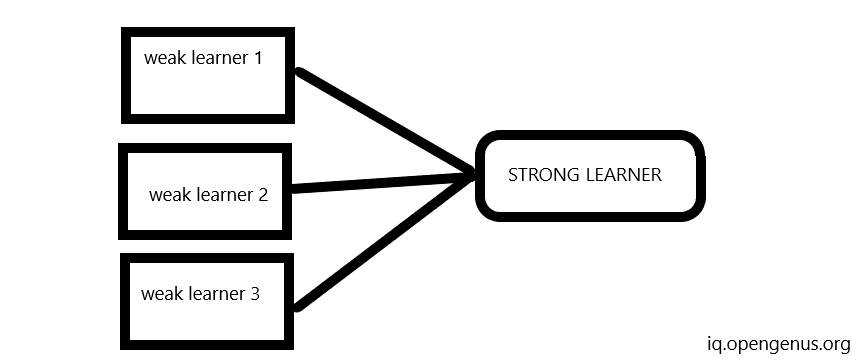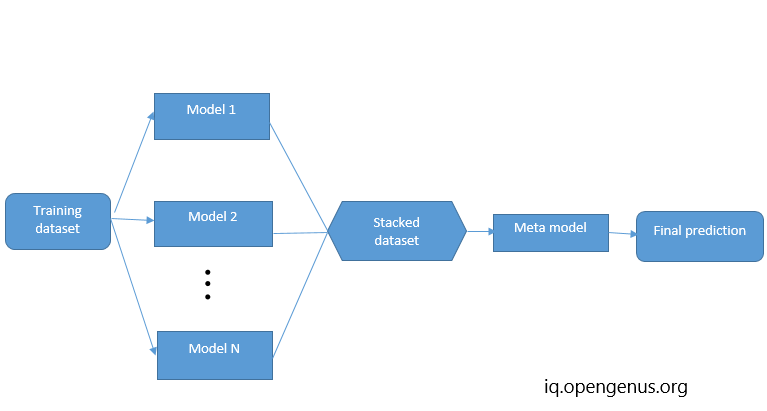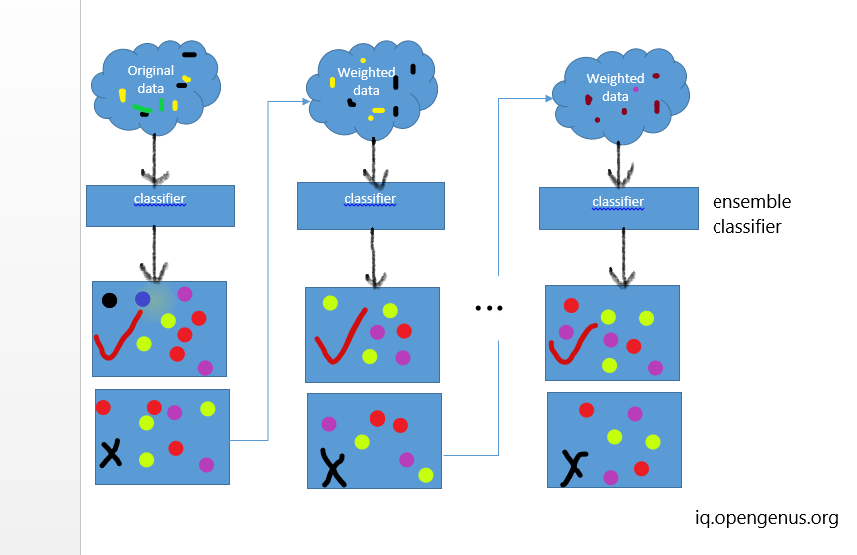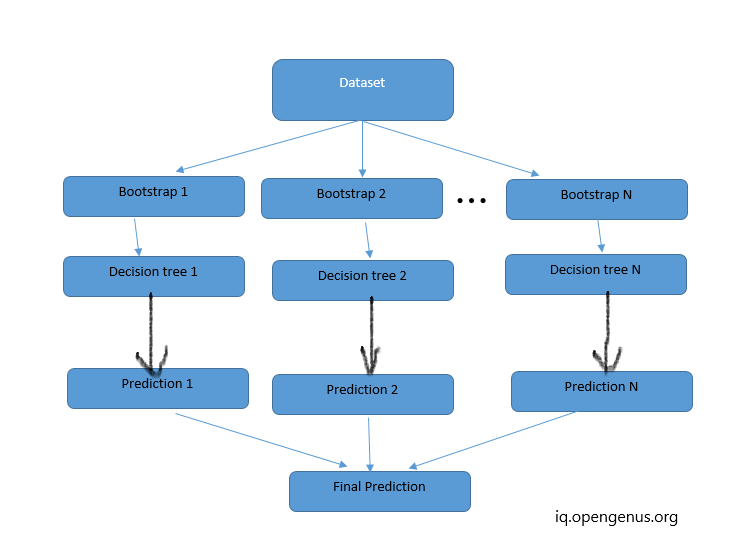×

Search anything:

# Introduction to Ensemble Learning

#### Machine Learning (ML)## CONTENTS

1. BOOSTING
2. BAGGING
3. STACKING

We have several classifier that classify our data well, but not great, and we had like to combine them into a super classifier that classify our data very well. This discipline is called Ensemble learning.#### Reason to combine many learners together

• Ensemble learning can improve the accuracy of machine learning models as it combines multiple models to make a final prediction. This can help to reduce the errors and biases present in individual models
• Ensemble learning can improve the generalization ability of machine learning models by reducing overfitting.
• Ensemble learning can help to handle complex data by combining multiple models that can deal with different aspects of the data.
• Ensemble learning can help to select the best model or combination of models for a particular problem by comparing the performance of different models.
• Ensemble learning can help to reduce the bias in machine learning models by combining the results of models that are trained on different datasets or with different algorithms.

#### Base learners

• Individual algorithms in the collection of machine learning algorithms are called base learners.
• When we generate multiple base-learners ,it should be reasonably accurate.
• Final accuracy when the base learners are combined are important rather than the accuracies of the base learners we started from.

Different ways of selecting base learners

1. Use different learning algorithms
2. Use different representation of the input object
3. Use the same algorithm with different parameters
4. Use different training sets to train different base learners

## Types of Ensemble Learning

Homogeneous and heterogeneous ensemble learning are two approaches to building ensemble models in machine learning.

In homogeneous ensemble learning, multiple instances of the same type of model are combined to create an ensemble model. For example, multiple decision trees or multiple support vector machines (SVMs) can be combined to create a stronger model. The idea is that by training multiple instances of the same model on different subsets of the training data or with different hyperparameters, the ensemble model can overcome the weaknesses of individual models and provide more accurate predictions.

• BOOSTING
• BAGGING

On the other hand, heterogeneous ensemble learning involves combining multiple types of models to create an ensemble model. For example, a decision tree can be combined with a neural network or an SVM to create a stronger model. Heterogeneous ensembles are usually more complex to build and require careful selection of different models that can complement each other's strengths and weaknesses. The advantage of heterogeneous ensembles is that they can provide better performance than a single model or a homogeneous ensemble.

• STACKING

## STACKING

Stacking, also known as stacked generalization, is an ensemble learning technique that combines multiple individual models to improve overall prediction performance. Unlike bagging and boosting, which combine models of the same type, stacking involves combining models of different types to leverage their unique strengths and weaknesses.

The basic idea behind stacking is to use a meta-model, often referred to as a blender or a meta-learner, to learn how to best combine the predictions of the individual models. The process of creating a stacking ensemble typically involves the following steps:

1. Splitting the training data into two or more disjoint subsets.
2. Training several base models on one subset of the data.
3. Using the trained base models to make predictions on the other subset of the data.
4. Using the predictions from step 3 as inputs to train a meta-model.
5. Using the trained meta-model to make predictions on new, unseen data.``````from sklearn.ensemble import RandomForestClassifier
from sklearn.linear_model import Logistic Regression
from sklearn.preprocessing import StandardScaler
from sklearn.pipeline import make_pipeline
from sklearn. ensemble import StackingClassifier
from sklearn.svm import LinearSVC
from sklearn.neural network import MLPClassifier
``````

## BOOSTING

Boosting is an ensemble learning method in which several weak models are combined to form a strong model. The process involves sequentially adding models to the ensemble, where each new model tries to correct the errors made by the previous models.

Here are the general steps for the working of boosting:

1. Train a weak model on the initial training data.
2. Evaluate the performance of the model on the training set and calculate the errors made by the model.
3. Increase the weight of the misclassified data points, so that they have a higher probability of being selected in the next iteration.
4. Train a new model on the updated training data, with higher weights for the misclassified data points.
5. Combine the predictions of the weak models using a weighted sum or another suitable method to obtain the final prediction.

This process is repeated for a fixed number of iterations or until the desired level of performance is achieved. Boosting algorithms, such as AdaBoost and Gradient Boosting, have been widely used in various machine learning tasks, including classification and regression.``````from sklearn.ensemble import AdaBoostClassifier
from sklearn.preprocessing import LabelEncoder
from sklearn.tree import DecisionTreeclassifier
import pandas as pd Import train test split function
``````

## BAGGING

Bagging is a technique used in ensemble learning to improve the stability and accuracy of machine learning models. It involves training multiple models independently on different subsets of the training data, and then aggregating their predictions to form a final output.

The bagging algorithm works as follows:

1. Random subsets of the training data are created with replacement, using a technique called bootstrap sampling. This means that some data points may be selected multiple times, while others may not be selected at all.
2. A base model is trained on each subset of the data. The base model can be any machine learning algorithm, such as a decision tree, support vector machine, or neural network.
3. Once all the base models have been trained, they are combined to make a final prediction. This can be done in several ways, such as taking the average of the predictions, or selecting the prediction with the most votes.

The idea behind bagging is that by training multiple models on different subsets of the data, we can reduce the variance of the final prediction. This is because each model is exposed to a different set of training examples, and so will have a slightly different view of the problem. By combining the predictions of multiple models, we can smooth out the variations in their individual predictions, and obtain a more robust and accurate overall result.``````from sklearn.datasets import make_classification
from sklearn. metrics importaccuracy_score
from sklearn.ensemble import BaggingClassifier
from sklearn.tree import DecisionTreeClassifier
from sklearn.svm import SVC
from sklearn.model_selection import train_test_split
``````

#### Sandra PS

My name is Sandra and I am a Student currently pursuing Masters in Computer Science with specialization in AI at Department of Computer Science University of Kerala.

Introduction to Ensemble Learning